# Pictographs

Pictographs

 1 Introduction to Pictographs 2 Important Notes on Pictographs 3 Advantages of Pictographs 4 Solved Examples on Pictographs 5 Challenging Questions on Pictographs 6 Practice Questions on Pictographs 7 Maths Olympiad Sample Papers 8 Frequently Asked Questions (FAQs)

We at Cuemath believe that Math is a life skill. Our Math Experts focus on the “Why” behind the “What.” Students can explore from a huge range of interactive worksheets, visuals, simulations, practice tests, and more to understand a concept in depth.

Book a FREE trial class today! and experience Cuemath’s LIVE Online Class with your child.

## Introduction to Pictographs

### Definition Of Pictograph:

"A pictograph is a representation of data using images or symbols."

Pictographs in maths are typically used in concepts like data handling.

They help in laying the foundation for data interpretation based on pictorial information.

Now after knowing pictograph definition, let us understand pictographs using a scenario.

A survey was conducted for 40 children by a fast food junction to understand the demand for different flavours of pizza available in their outlet.

The results were as follows: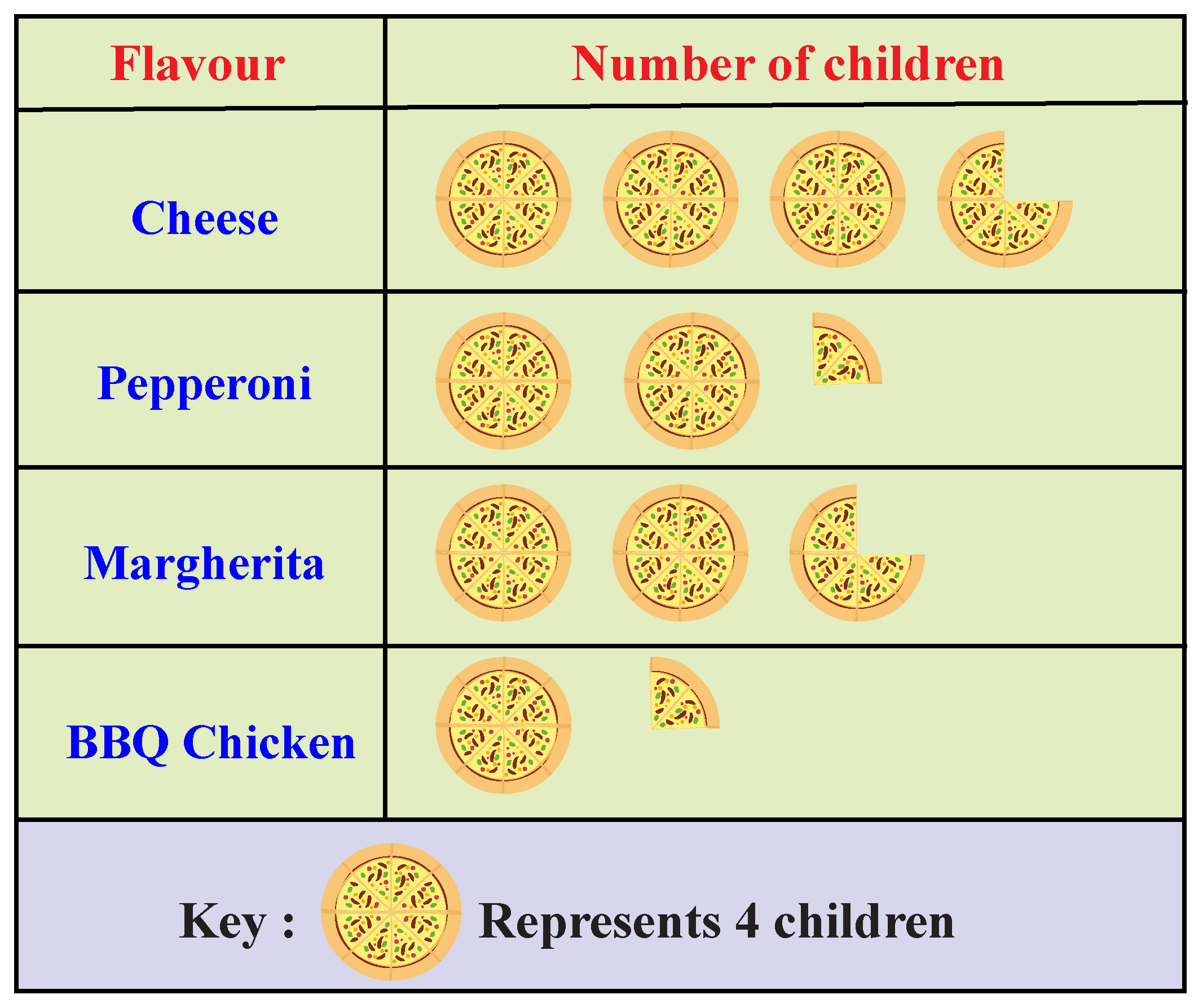Can you identify the most loved flavour by observing the above table?

If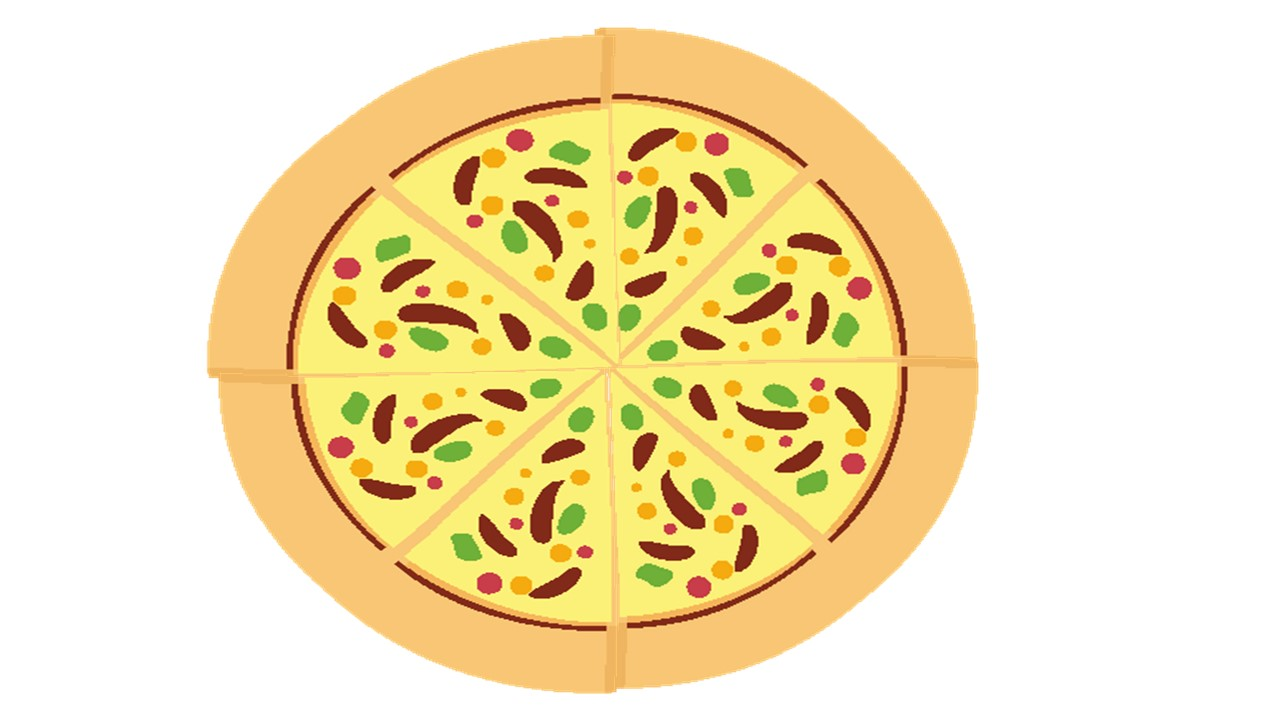represents 4 children, then what would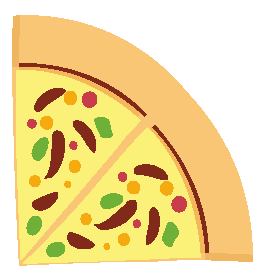represent?

Let's try to find the answer by watching this simulation.

The scenario that we discussed above represents information in a pictographic manner.

Here, the symbolis used to represent data (i.e. the number of students).

We need to do simple math to understand how many children voted for each of the flavours.

Multiply the number of symbols for the given flavour with the value of each symbol.

For example, the number of children who liked Pepperoni  \begin{align} &=2\dfrac{1}{4}\times 4\\&= 2\times 4 +\dfrac{1}{4}\times 4\\&=8+1=9. \end{align}

We use a key to denote the value of the symbol.Important Notes
1. All the symbols/icons must be of the same size.
2. A fraction of an icon can also be used to show data.

Thus, after going through the above pictograph example, we come to the basic formula which is used to find the value of the data of the categories given in the chart/table.

Value of a category = Product of the number of times the symbol is used and the value of each symbol.

If we denote the number of times by $$N$$, and the value of each symbol by $$S$$, we get:

 $$\text{Value of a category}= N \times S$$

Let's solve some more pictograph examples.

Example:

As schools are closed and meeting people outside is prohibited due to lockdown, children are being forced to stay indoors all day.

A survey was conducted by a local school for students of grade 6 on how they spend their time on a weekday.

The average duration per child was calculated and the outcomes were recorded in the form of the following pictorial graph.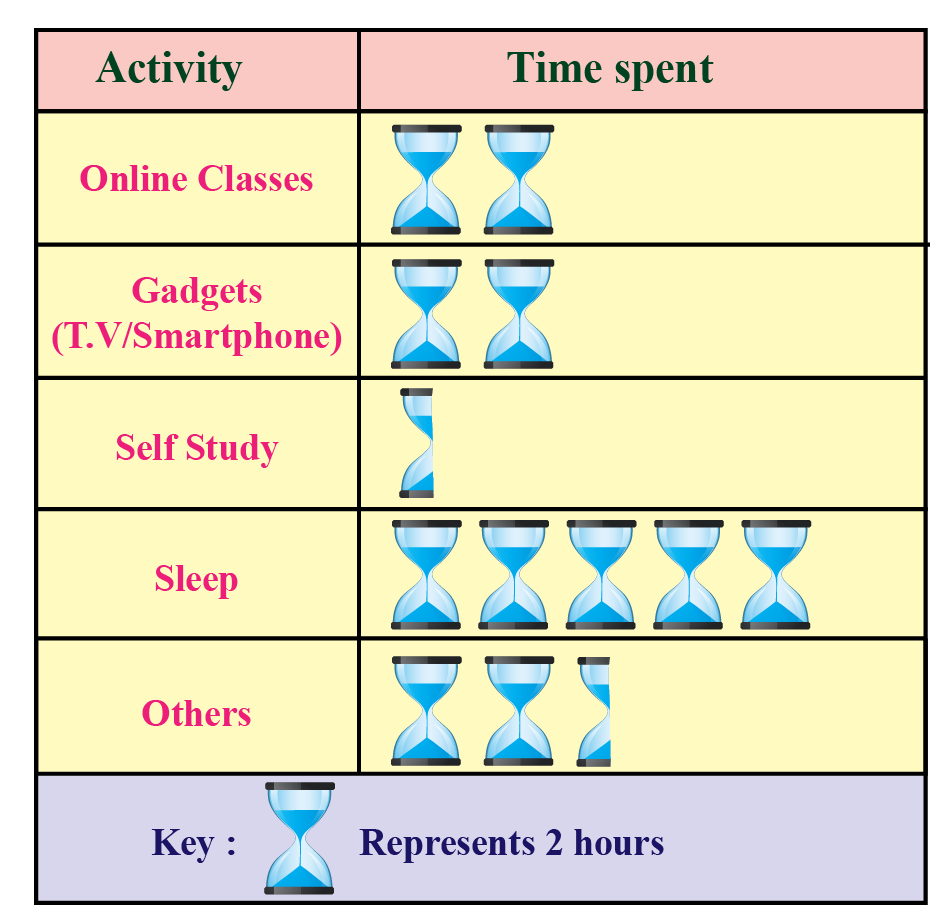Based on the observations, answer the following questions.

1. What is the average time spent by a child on gadgets?

2. How many hours on an average does a child sleep during the lockdown on a weekday?

3. What is the total average time spent by a child on academics?

4. How many hours are spent on other activities?

1. The average time spent by an average child on gadgets $$2 \times 2 = 4\, hours$$.

2. An average child sleeps for  $$5\times 2 = 10\, hours\,$$ on a weekday during the lockdown.

3. Total time taken for academics $$= 2 \times 2 + \dfrac{1}{2}\times 2= 4+1=5\, hours$$.

4. Time spent on other activities  $$= 2 \times 2 + \dfrac{1}{2}\times 2= 4+1=5 \,hours$$.

Want to understand the “Why” behind the “What”? Explore Pictographs with our Math Experts in Cuemath’s LIVE, Personalised, and Interactive Online Classes.

Make your kid a Math Expert, Book a FREE trial class today!

Pictographs are widely used in maths.
There are several advantages to using pictographs in maths.
These are some of the advantages.
• They can be used primarily for making early learners associate objects with numbers.
• They help in visually formatting statistics.
• They make data visually interesting and easy to understand.
• Representing data pictographic way can be useful for representing a large amount of data.

Also referred to as “pictograms” or ” picture charts”, pictographs in maths are of great help when we want to make data more engaging.

Now, let's do a small activity.

Let's create a pictograph with the information given below.

In Mrs. Mary's class, math tests were conducted in the first term.

If a student gets an "A" in their math tests, a star will be placed next to their name on the class chart.

Whoever gets the most stars by the end of two months will get pizza for lunch.

In the first month, Kate got 5 stars, Carol got 3 stars, and Tracie got 2 stars.

In the second month, Kate got 2 stars, Carol got 1 star, and Tracie got 4 stars.

Drag the stars given below and drop them in the column in the pictograph against their names to show how many stars each student received.

Can you now identify who received a pizza at lunch from Mrs. Mary at the end of two months?

You are right!

Kate got a pizza at lunch for getting the most number of stars in the math tests.

Help your child score higher with Cuemath’s proprietary FREE Diagnostic Test. Get access to detailed reports, customised learning plans, and a FREE counseling session. Attempt the test now.

## Solved Examples on Pictograph

 Example 1

The table below is in the form of a pictorial graph.
It shows the quantity of apples of different varieties (in Kilogram) being sold at a store in a given month.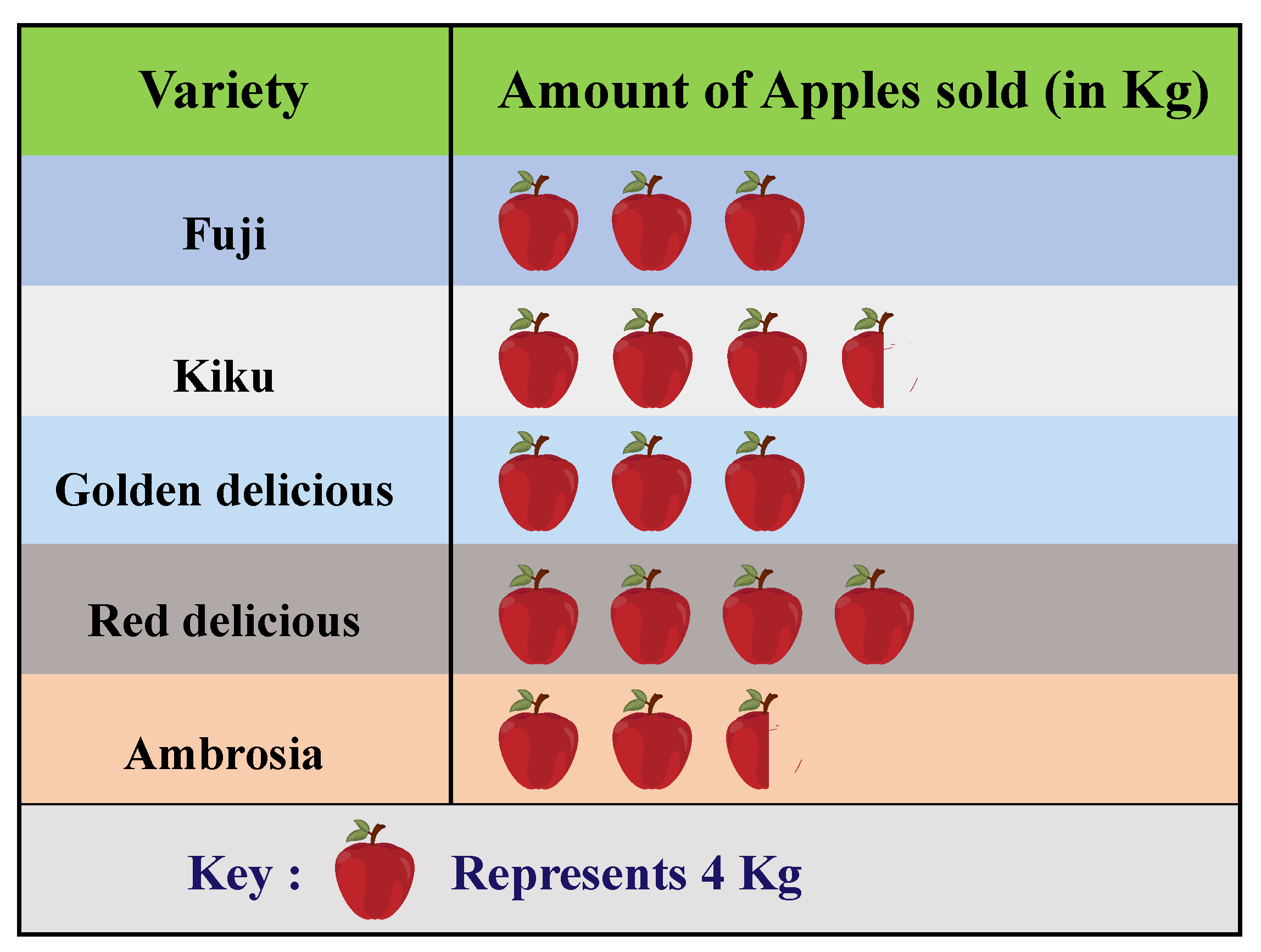Based on the pictograph, answer the following questions.

(a) Which variety of apple is the best seller?

(b) How many more kilograms of Fuji apples were sold compared to Ambrosia?

(c) What is the total quantity of apples sold by the store?

Solution

(a) Red Delicious is the best seller.

(b) \begin{align} \text{Quantity of Fuji} &=3 \times 4= 12\,kg\\\text {Quantity of Ambrosia}&=2 \dfrac{1}{2}\times 4\\&=2 \times 4 + \dfrac{1}{2}\times 4\\&=8+2=10\,kg. \\ \text{Difference} &= 12-10= 2 \,kg.\end{align}

(c) Total quantity of apples sold by the store $$= 16\times 4=64 \,kg.$$

 Example 2

The following table shows information about the modes of transport used by students to commute to school.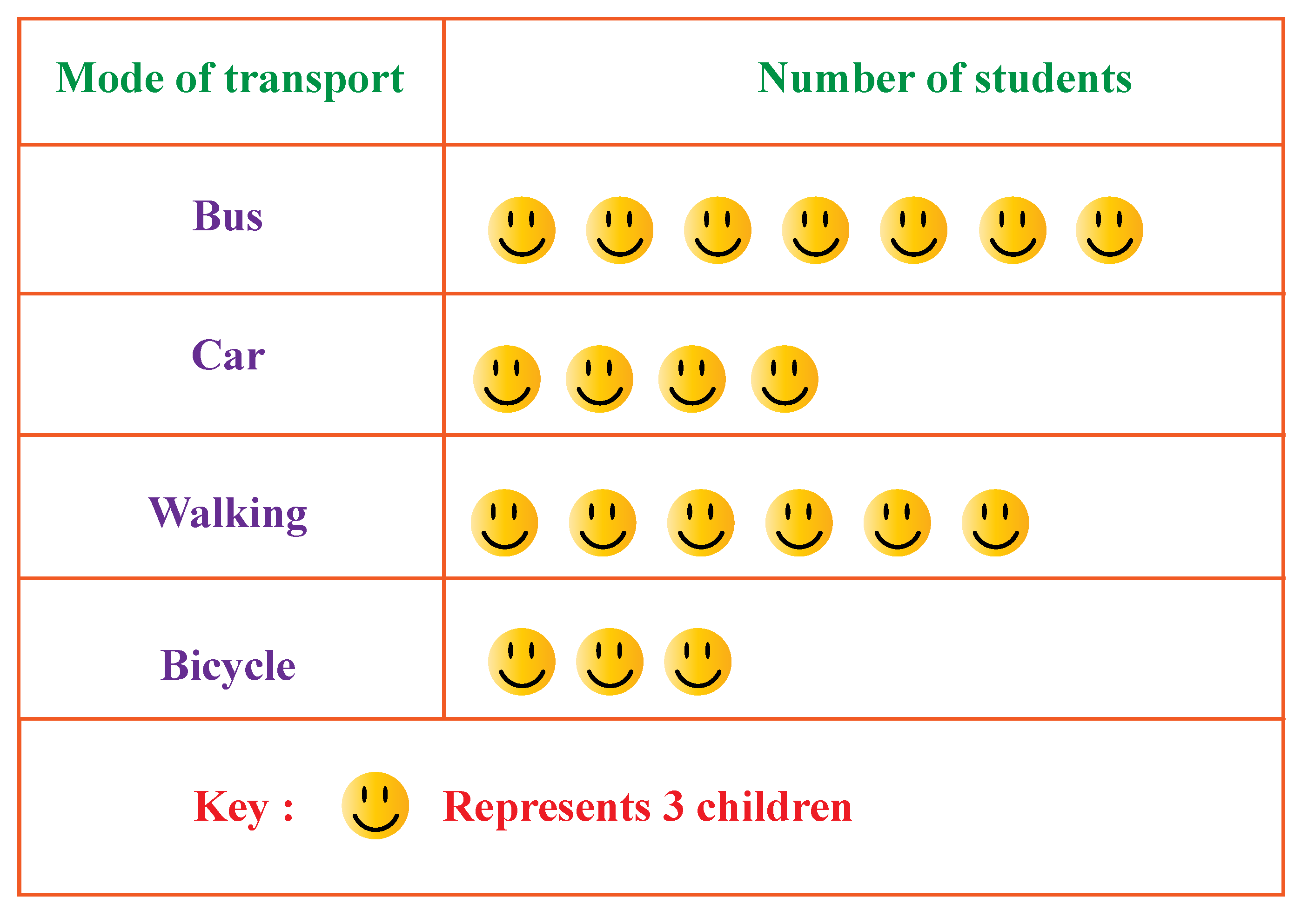Answer the following questions based on the information given above.

1. How many students commute by car?

2. Which is the most commonly used mode of transport?

3. Which is the least preferred mode to reach school?

Solution

Answer 1: No. of students who commute by car$$=4 \times 3=12.$$

Answer 2: The bus is the most commonly used mode of transport.

Answer 3: The least preferred mode to reach school is a bicycle.

 Example 3

The following pictograph shows the number of bananas and oranges consumed by students of three schools in a locality.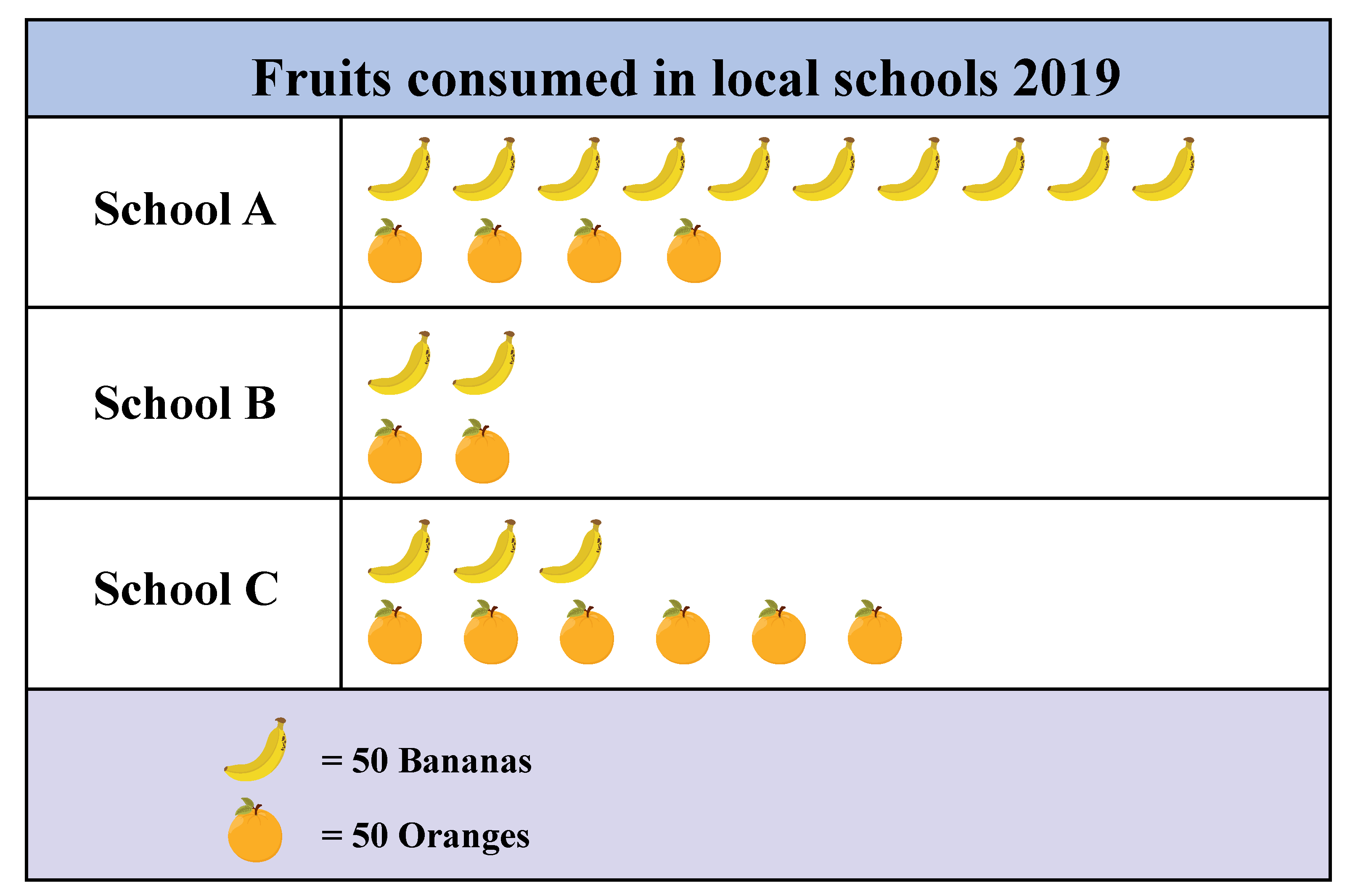Based on the above information, answer the following questions.

1. In which school the total number of bananas consumed is more than oranges?

Answer: In school A, the total number of bananas consumed is more than oranges.

2. What is the difference between the number of oranges consumed by students of schools A and C?

Answer: Number of oranges consumed in school A $$= 4 × 50 = 200$$

Number of oranges consumed in school C  $$= 6 × 50 = 300$$

Difference $$= 300- 200.= 100$$

3. In which school the number of bananas and oranges consumed is the same?

Answer: In school B, the number of bananas and oranges consumed is the same.

4. How many fruits were consumed by children of school C?

Answer: Number of fruits consumed by children of school C
$$=$$ No. of bananas + No. of oranges.

$$=3 \times 50 + 6 \times 50 = 150 + 300 = 450$$Challenging Questions
Four friends are playing with 44 pieces of puzzle. The number of pieces that each of them has is recorded in the form of a pictograph below.
But there is some information missing. Can you find the missing entries using the given hints?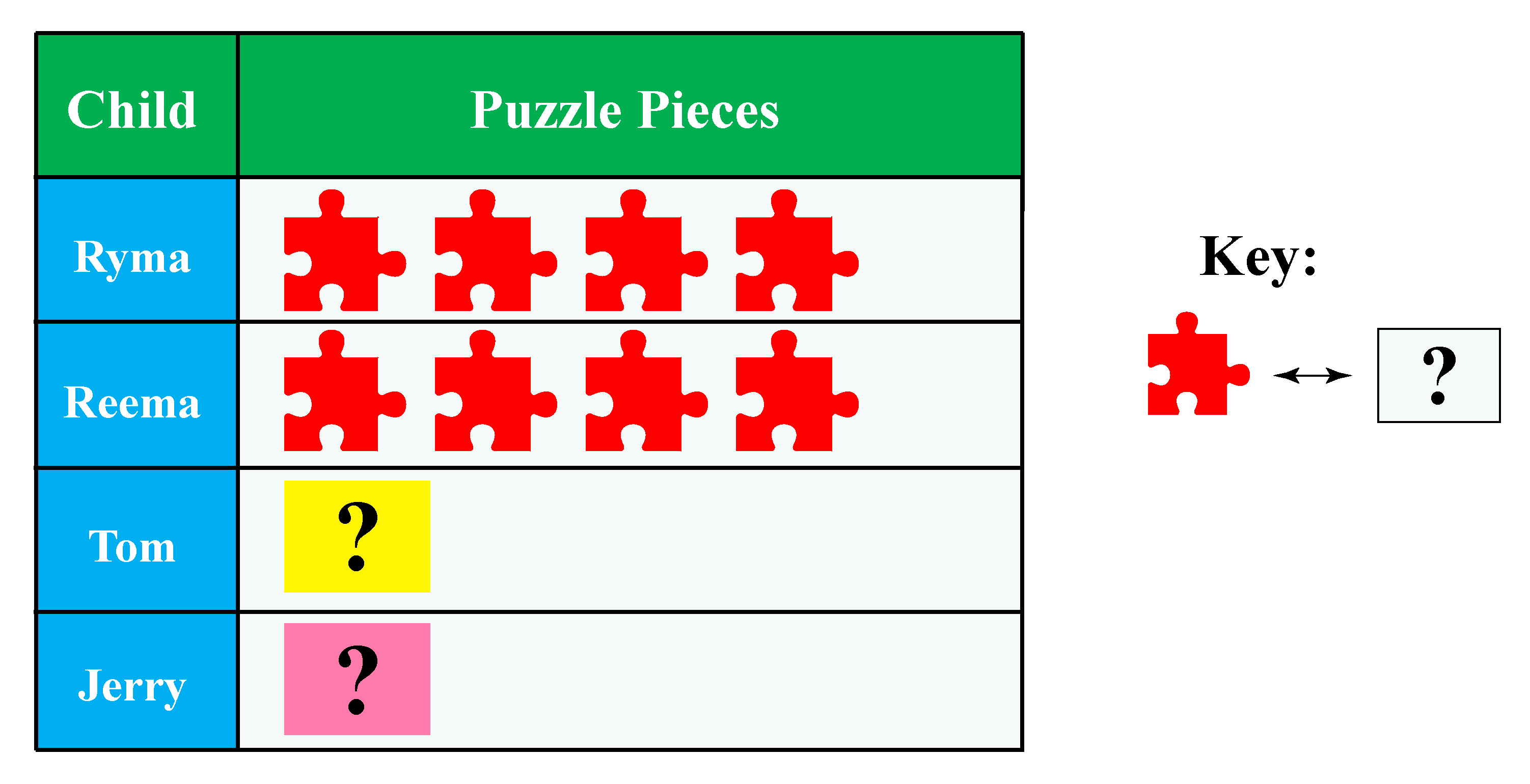Q1. 1 icon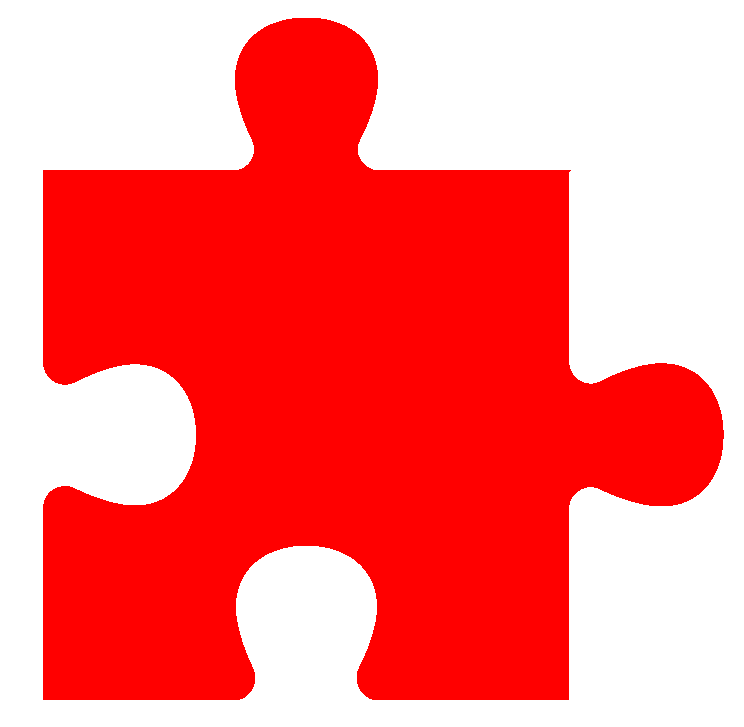= ________.    Q2. How many pieces does Tom and Jerry have?

## Practice Questions

Here are a few activities for you to practice. Select/Type your answer and click the "Check Answer" button to see the result.

IMO (International Maths Olympiad) is a competitive exam in Mathematics conducted annually for school students. It encourages children to develop their math solving skills from a competition perspective.

## 1. What is a pictograph? Give examples.

A pictograph is a representation of data using images or symbols.

We see these pictographs in many real-life situations.

A simple example is the star rating that is given by customers for items purchased.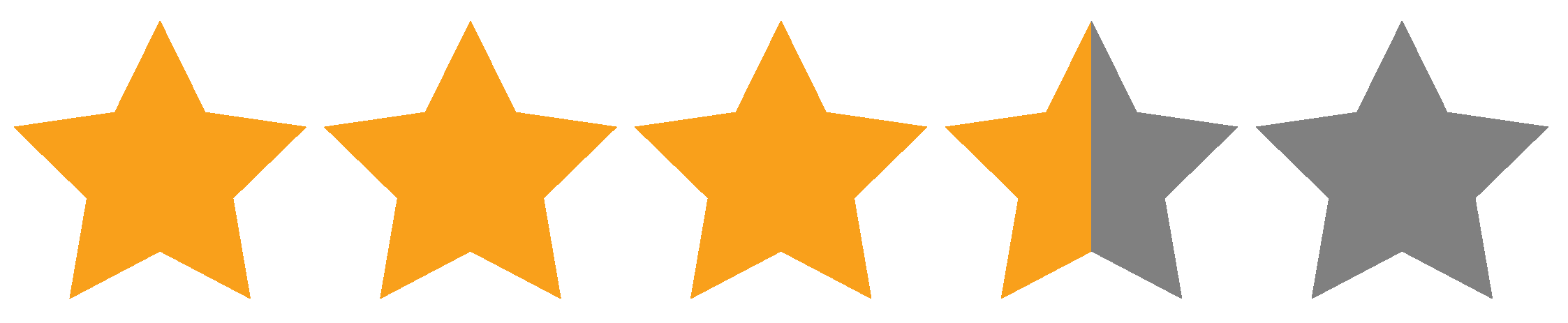In the picture above, if it is given that 1 star = 20 points, then the rating by the customer will be
20 x 3.5 = 70 out of 20 x 5 = 100

## 2. What is a pictograph chart?

A pictograph chart is a chart that uses icons or symbols to represent information or data.

## 3. What is a pictograph used for?

A pictograph is used to represent data in the form of pictures or icons which makes the presentation more interesting and easier to understand.

More Important Topics
Numbers
Algebra
Geometry
Measurement
Money
Data
Trigonometry
Calculus
More Important Topics
Numbers
Algebra
Geometry
Measurement
Money
Data
Trigonometry
Calculus
Learn from the best math teachers and top your exams

• Live one on one classroom and doubt clearing
• Practice worksheets in and after class for conceptual clarity
• Personalized curriculum to keep up with school